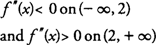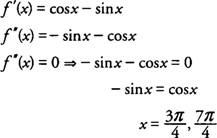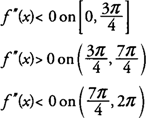## Concavity and Points of Inflection

The second derivative of a function may also be used to determine the general shape of its graph on selected intervals. A function is said to be concave upward on an interval if f″(x) > 0 at each point in the interval and concave downward on an interval if f″(x) < 0 at each point in the interval. If a function changes from concave upward to concave downward or vice versa around a point, it is called a point of inflection of the function.

In determining intervals where a function is concave upward or concave downward, you first find domain values where f″(x) = 0 or f″(x) does not exist. Then test all intervals around these values in the second derivative of the function. If f″(x) changes sign, then ( x, f(x)) is a point of inflection of the function. As with the First Derivative Test for Local Extrema, there is no guarantee that the second derivative will change signs, and therefore, it is essential to test each interval around the values for which f″(x) = 0 or does not exist.

Geometrically, a function is concave upward on an interval if its graph behaves like a portion of a parabola that opens upward. Likewise, a function that is concave downward on an interval looks like a portion of a parabola that opens downward. If the graph of a function is linear on some interval in its domain, its second derivative will be zero, and it is said to have no concavity on that interval.

Example 1: Determine the concavity of f(x) = x 3 − 6 x 2 −12 x + 2 and identify any points of inflection of f(x).

Because f(x) is a polynomial function, its domain is all real numbers.Testing the intervals to the left and right of x = 2 for f″(x) = 6 x −12, you find thathence, f is concave downward on (−∞,2) and concave upward on (2,+ ∞), and function has a point of inflection at (2,−38)

Example 2: Determine the concavity of f(x) = sin x + cos x on [0,2π] and identify any points of inflection of f(x).

The domain of f(x) is restricted to the closed interval [0,2π].Testing all intervals to the left and right of these values for f″(x) = −sin x − cos x, you find thathence, f is concave downward on [0,3π/4] and [7π/4,2π] and concave upward on (3π/4,7π/4) and has points of inflection at (3π/4,0) and (7π/4,0).

Back to Top
A18ACD436D5A3997E3DA2573E3FD792A